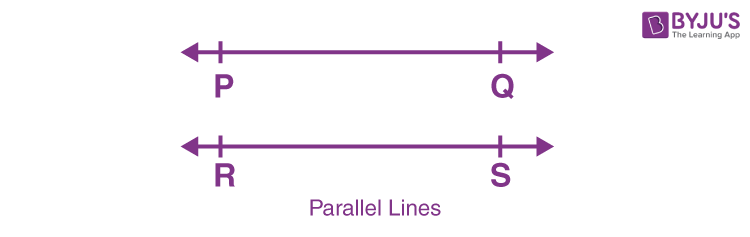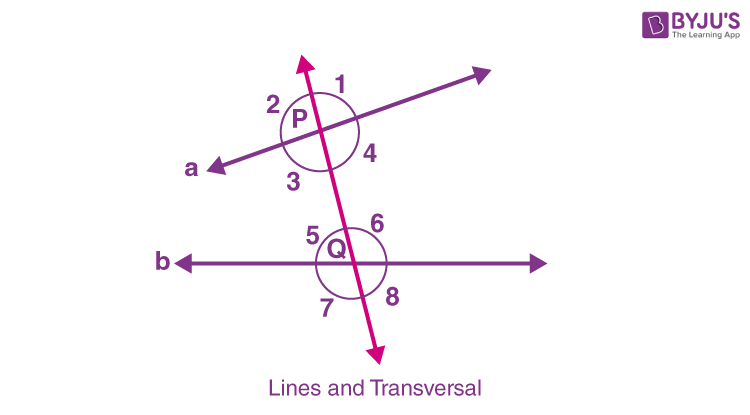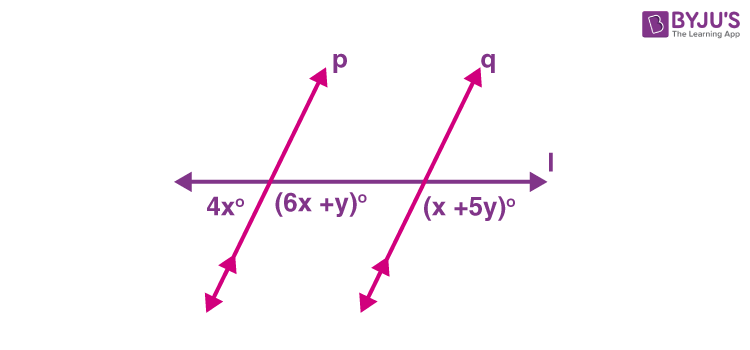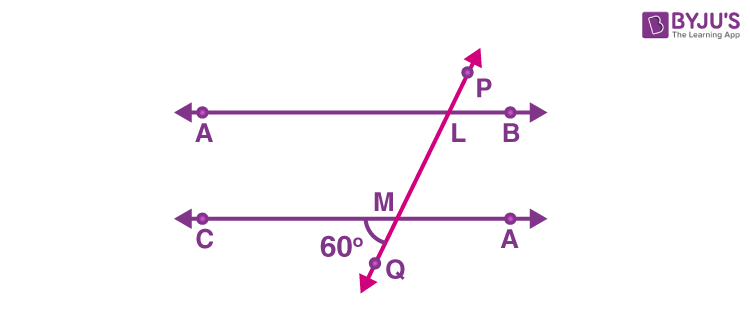# Parallel Lines and Angle Relationships

Parallel lines are the lines that do not intersect or meet each other at any point in a plane. They are always parallel and are at equidistant from each other. Parallel lines are non-intersecting lines. We can also say Parallel lines meet at infinity.

Also, when a transversal intersects two parallel lines, then pairs of angles are formed, such as:

• Corresponding angles
• Alternate interior angles
• Alternate exterior angles
• Vertically opposite angles
• Linear pair

If two lines are intersecting each other at a point, in a plane, they are called intersecting lines. If they meet each other at 90 degrees, then they are called perpendicular lines.

## Definition

Two lines are said to be parallel when they do not meet at any point in a plane. Lines which do not have a common intersection point and never cross path with each other are parallel to each other. The symbol for showing parallel lines is ‘||’.

Two lines which are parallel are represented as:

$$\begin{array}{l}\overleftrightarrow{AB}||\overleftrightarrow{CD}\end{array}$$

This means that line AB is parallel to CD.

The perpendicular distance between the two parallel lines is always constant.In the figure shown above, the line segments PQ and RS represent two parallel lines as they have no common intersection point in the given plane. Infinite parallel lines can be drawn parallel to lines PQ and RS in the given plane.

## Pairs of Angles

Lines can either be parallel or intersecting. When two lines meet at a point in a plane, they are known as intersecting lines. If a line intersects two or more lines at distinct points then it is known as a transversal line.

In figure 2, line l intersects lines a and b at points P and Q respectively. The line l is the transversal here.

∠1,∠2,∠7 and ∠8 are the exterior angles and ∠3,∠4,∠5 and ∠6 denote the interior angles.
The angle pairs formed due to intersection by a transversal are named as follows:

1. Corresponding Angles: ∠1 and ∠6; ∠4 and ∠8; ∠2 and ∠5; ∠3 and ∠7 are the corresponding pair of angles.
2. Alternate Interior Angles: ∠4 and ∠5 ; ∠3 and ∠6 denote the pair of alternate interior angles.
3. Alternate Exterior Angles: ∠1 and ∠7; ∠2 and ∠8 are the alternate exterior angles.
4. Same side Interior Angles: ∠3 and ∠5; ∠4 and ∠6 denote the interior angles on the same side of the transversal or co-interior or consecutive interior angles.If the lines a and b are parallel to each other as shown, then the following axioms are given for angle pairs of these lines.

## Properties of Parallel Lines

As we have already learned, if two lines are parallel, they do not intersect, on a common plane. Now if a transversal intersects two parallel lines, at two distinct points, then there are four angles formed at each point. Hence, below are the properties of parallel lines with respect to transversals.

• Corresponding angles are equal.
• Vertical angles/ Vertically opposite angles are equal.
• Alternate interior angles are equal.
• Alternate exterior angles are equal.
• Pair of interior angles on the same side of the transversal are supplementary.

#### For More Information On Parallel Lines and Intersecting Lines, Watch The Below Video:## Parallel Lines Axioms and Theorems

Go through the following axioms and theorems for the parallel lines.

### Corresponding Angle Axiom

If two lines which are parallel are intersected by a transversal then the pair of corresponding angles are equal.

From Fig. 3: ∠1=∠6, ∠4=∠8, ∠2= ∠5 and ∠3= ∠7

The converse of this axiom is also true according to which if a pair of corresponding angles are equal then the given lines are parallel to each other.

### Theorem 1

If two lines which are parallel are intersected by a transversal then the pair of alternate interior angles are equal.

From Fig. 3: ∠4=∠5 and ∠3=∠6

Proof: As, ∠4=∠2 and ∠1=∠3(Vertically Opposite Angles)

Also, ∠2=∠5 and ∠1=∠6 (Corresponding Angles)

⇒∠4=∠5 and ∠3=∠6

The converse of the above theorem is also true which states that if the pair of alternate interior angles are equal then the given lines are parallel to each other.

### Theorem 2

If two lines which are parallel are intersected by a transversal then the pair of interior angles on the same side of the transversal are supplementary.

∠3+ ∠5=180° and ∠4+∠6=180°

As ∠4=∠5 and ∠3=∠6 (Alternate interior angles)

∠3+ ∠4=180° and ∠5+∠6=180° (Linear pair axiom)

⇒∠3+ ∠5=180° and ∠4+∠6=180°

The converse of the above theorem is also true which states that if the pair of co-interior angles are supplementary then the given lines are parallel to each other.

### Applications of Parallel Lines in Real Life

One will be able to see lines which are parallel to each other in real life too if only one has the patience and is observant enough to do so. For instance, take the railroads. The railway tracks are literally parallel lines. The two lines or tracks are meant for the wheels of the train to travel along on. The difference between the parallel lines imagined by mathematicians and the ones who actually make the railway tracks is that mathematicians have the liberty to imagine the parallel lines over flat surfaces and paper, while trains travel across all sorts of terrain, from hills, slopes and mountains to over bridges.

According to mathematicians when two parallel lines are graphed, they must always be at the same angle, which means they’ll have the same slope or steepness.

## Solved Examples

Q.1: In the given figure, p || q and l is a transversal. Find the values of x and y.Solution: Since, 6x+y and x+5y are corresponding angles.

6 x + y = x + 5 y

6 x – x = 5 y – y

5 x = 4 y

x = 4y/5

Now, 4x and 6x+y are linear pair of angles, so,

4 x + 6 x + y = 180°

10x + y = 180°

40y/5 + y = 180°

45y/5 = 180°

45y = 180  × 5 = 900°

y = 20

x = (4 × 20)/5 = 16

Therefore, x = 16 and y = 20

Q.2:  In Figure, AB and CD are parallel lines intersected by a transversal PQ at L and M respectively, If ∠CMQ = 60, find all other angles in the figure.Solution:

∠ALM = ∠CMQ = 60° [corresponding angles]

∠LMD = ∠CMQ = 60° [Vertically opposite angles]

∠ALM = ∠PLB = 60  [Vertically opposite angles]

Here, ∠CMQ + ∠QMD = 180° are the linear pair

∠QMD = 180° – 60° = 120°

Now,

∠QMD = ∠MLB = 120° [Corresponding angles ]

∠QMD = ∠CML = 120°  [Vertically opposite angles]

∠MLB = ∠ALP = 120° [Vertically opposite angles]

## Frequently Asked Questions – FAQs

Q1

### What are parallel lines?

Parallel lines are those lines on a plane that do not meet each other at any point. They are non-intersecting lines.
Q2

### What are the properties of parallel lines?

Parallel lines are always equidistant apart from each other. They do not intersect each other. When cut by a transversal, parallel lines form a pair of angles. Hence, corresponding angles are equal, alternate interior angles are equal, alternate exterior angles are equal, vertical angles are equal and sum of interior angles on the same side of transversal are supplementary.
Q3

### If x and y are pair of interior angles on the same side of transversal and x is equal to y, then what are the angles?

Given, x and y are pairs of interior angles on the same side of transversal, hence they are supplementary.
x + y = 180°
Also, x and y are equal. Therefore,
x + x = 180°
2x = 180°
x = 90°
Therefore the angles are equal to 90 degrees.
Q4

### If one of the angle is 45 degrees, then its corresponding angle will be?

If one of the pair of angles is 45 degrees, then its corresponding angle is also equal to 45 degrees.
Q5

### If one of the angles is 108 degrees, then its vertically opposite angle is?

If one of the pairs of angles is 108 degrees, then its vertically opposite angle is also equal to 108 degrees.
Test your knowledge on Parallel lines